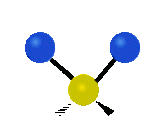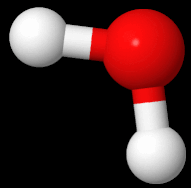# 5.1: Energy

$$\newcommand{\vecs}{\overset { \rightharpoonup} {\mathbf{#1}} }$$ $$\newcommand{\vecd}{\overset{-\!-\!\rightharpoonup}{\vphantom{a}\smash {#1}}}$$$$\newcommand{\id}{\mathrm{id}}$$ $$\newcommand{\Span}{\mathrm{span}}$$ $$\newcommand{\kernel}{\mathrm{null}\,}$$ $$\newcommand{\range}{\mathrm{range}\,}$$ $$\newcommand{\RealPart}{\mathrm{Re}}$$ $$\newcommand{\ImaginaryPart}{\mathrm{Im}}$$ $$\newcommand{\Argument}{\mathrm{Arg}}$$ $$\newcommand{\norm}{\| #1 \|}$$ $$\newcommand{\inner}{\langle #1, #2 \rangle}$$ $$\newcommand{\Span}{\mathrm{span}}$$ $$\newcommand{\id}{\mathrm{id}}$$ $$\newcommand{\Span}{\mathrm{span}}$$ $$\newcommand{\kernel}{\mathrm{null}\,}$$ $$\newcommand{\range}{\mathrm{range}\,}$$ $$\newcommand{\RealPart}{\mathrm{Re}}$$ $$\newcommand{\ImaginaryPart}{\mathrm{Im}}$$ $$\newcommand{\Argument}{\mathrm{Arg}}$$ $$\newcommand{\norm}{\| #1 \|}$$ $$\newcommand{\inner}{\langle #1, #2 \rangle}$$ $$\newcommand{\Span}{\mathrm{span}}$$$$\newcommand{\AA}{\unicode[.8,0]{x212B}}$$

Learning Objectives

• Define energy and work
• Define basic terms of thermodynamics like system and surroundings
• Describe the nature of energy transfers
• Compare and contrast exothermic and endothermic processes sign conventions associated with these process.

## Introduction

In this Chapter we will introduce thermodynamics, the science of energy and its transformations. Energy can be considered to be the capacity to do work or transfer heat.

Definition: Energy

Energy is the capacity to do work (w) or transfer heat (q)

In Chapter 1 we introduced the concepts of kinetic and potential energy, and we will now take a deeper look at these. From a macroscopic perspective we sense objects as hot or cold as represented by the temperature, and recognize that heat will flow from hot to cold, but often mistake this to mean temperature is energy, which it is not. Thermal energy can be related to the random motion of molecules as they vibrate, rotate and translate in space.

## Molecular Kinetic Energy

### Translational Kinetic Energy

$$E_{k} = \frac{1}{2}mv^{2}$$, is the energy associated with the movement of a chemical entity's center of mass, where m is the mass of the chemical entity (molecule, atom or ion) and v is the velocity of its center of mass. In the fluid phases (gas and liquids) there will be a distribution of translational energies as different molecules randomly move around each other.

### Vibrational Kinetic Energy

$$E_{k} = \frac{1}{2}kx^{2}$$, is the energy associated with atom's vibrational motion, which can be modeled as a oscillating spring with k being Hooke's law constant and x is the displacement from the equilibrium location. That is, even the atoms in a solid, be it a covalent bond or ionic bond (crystal lattice) are not static, and they vibrate back and forth around equilibrium positions like in a spring, with the the average distance being the "bond distance". Note, atoms oscillate because there are attractive and repulsive forces acting on them which change as their location changes. For example, in the diatomic hydrogen bond, the nuclei are accelerated towards each other if the attractive forces are greater than the repulsive, but as they get closer and closer, the nuclear/nuclear repulsion becomes stronger, and they start to be repulsed. As they move farther and farther apart, these forces become weaker, and the attractive forces once again begin to dominate, causing them to approach each other, with the resulting motion like the oscillation of a spring. Hooke's law equation actually describes the potential energy of the oscillator, when the distance is a maximum from the equilibrium, but as energy is conserved, this is equal to the kinetic energy that atoms feel in the midpoint of the oscillation. The hotter a substance the faster the vibrational frequency.Figure $$\PageIndex{1}$$: Three fundamental vibrational modes for water, images from Wikipedia article on Electromagnetic absorption by water.

### Rotational Kinetic Energy

$$E_{\omega} = \frac{1}{2}I\omega^{2}$$ , is the energy of a chemical entity associated with rotation, where $$\omega$$ is the rotational frequency (how fast the molecule spins) and I is the moment of inertia (in a sense, its resistance to spinning, the farther apart the hydrogen the greater I, the closer together, the smaller I. There can be multiple axis of rotation.Figure $$\PageIndex{2}$$: Water molecule spinning along axis that bisects H-O-H angle. (CC BY-SA 4.0 Internation; Darekk2 via Wikipedia)

### Molecular Potential Energy

Coulombic Energy is the energy between charged particles. There are both repulsive forces (between like charged particles) and attractive forces (between opposite charged particles).Figure 5.1.3 image showing repulsion of like charges and attraction of opposite

### Atomic Coulombic Energy

The coulombic energy of attraction of the electron to the nucleus of an atom. These are weakest for outer shell electrons and strongest for core electrons.

### Bond Coulombic Energies

The energies of covalent and ionic bonds. In the case of ionic compounds they are the attractive and repulsive interactions of the various cations and anions. For covalent, they are the +/- attractions of electrons to nuclei of different atoms, but there are also repulsive interactions of the -/- electrons and +/+ nuclei. The equilibrium distance is considered as the potential energy of the bond. As bonds are broken and formed during a chemical reaction energy the potential energy may be released or absorbed from the surroundings which either gain or lose kinetic energy.

### Nuclear Energy

There are "strong" and "weak" forces holding the nucleus together and these will not be covered in this class.

Exercise $$\PageIndex{1}$$

Identify the type of energy described by each of the following statements as potential or kinetic

1. Vibrational motion of atoms in a bond that are stimulated by infrared radiation
2. Energy of an electron in an atomic orbit
3. Energy of a covalent bond
4. Rotation of a molecule induced by microwave radiation

Kinetic

Potential

Potential

Kinetic

## Units of Energy

Work based definition of energy

This is the SI unit of energy and one Joule is a Newton meter (J = N.m), where a Newton is the SI unit of force. The Newton (named after Isaac Newton) comes from classical mechanics and Newton's second Law of motion, F = ma (mass times acceleration), and acceleration is the change in velocity per unit time, $$a=\frac{\Delta v}{\Delta t}$$ and velocity is the vectorial change in displacement per unit time, $$v=\frac{\Delta x}{\Delta t}$$ , where x is the displacement in a specific direction. So like any derived SI unit, the Joule can be described in terms of the SI base units, $$1J=1kg\frac{m^{2}}{sec^{2}}$$

### Calorie

Heat based definition of energy

Historically, the calorie is the energy required to raise one gram of water one degree celsius (from 14.5oC to 15.5oC), but today the calorie is considered to be a depreciated unit, and according to NIST Special Publication 1038, "The International System of Units (SI)-Conversion Factors of General Use", the definition of the calorie is based on the Joule:

1cal ≡ 4.184 Joules

Note, the above is a defined number and should not be treated as if it has significant digits. Also, the calorie is actually a very small unit of measurement and it should be noted that the nutritional Calorie (written with a capital C) is really a kilocalorie.

Exercise $$\PageIndex{2}$$

The NIH provides a table of Estimated Calorie Requirements (kilocalories) for gender and age groups that indicates an active 19-30 year old male should needs around 3,000. calories. How many joules is this? Use appropriate SI Prefix in your answer.

12.55MJ

## What is Work?

Work has many forms, of which mechanical(translational) work can be described as the energy used to displace an object a distance d when opposed against a force, like that of gravity.

$w=Fd$

where

• $$w$$ is the work
• $$F$$ is the opposing force
• $$d$$ is the distance the object was displaced.

Note, there are other types of work, like the expansion of a gas and electric work (the work done to move a charged particle in an electric field). In this class we will mostly be interested in PV work, which can be visualized as the work done through the translational motion of molecules moving in three dimensional space.

### Work of Expansion: Pressure-Volume (PV) Work

To fully understand this we need to have covered gasses, but we can imagine molecules in a gas as having translational motion, which could do work against the surroundings. If we could imagine a frictionless piston in a cylinder there would be three possibilities where the pressure inside is either equal to, greater than or less than the pressure outside. If the pressure inside is greater, the gas inside will expand moving the piston and doing work on the surroundings. If the pressure outside is greater, the piston will move inward compressing the gas inside as work is done on the system.

$$P_{ext} = P_{int}$$ The system is at equilibrium, no work is done
$$P_{ext} > P_{int}$$ The system contracts and work is done on the system
$$P_{ext} < P_{int}$$ The system expands and work is done on the environmentFigure 5.1.4 PV Work. Using a frictionless piston, if the external pressure is less than Pint (a), the gas inside the piston will expand, forcing the piston to perform work on its surroundings. The final volume (Vf) will be greater than Vi. Alternatively, if the external pressure is greater than Pint (b), the gas will be compressed, and the surroundings will perform work on the system.

Pressure is defined as Force per unit area $$P = \dfrac{F}{A}$$, so F=PA, and if the external pressure in Figure 5.1.5 is greater than the internal pressure, work will be done on the system, causing it to contract by the volume AΔh, so ΔV = AΔh

$w = F d = P_{ext}AΔh$

The change in the volume of the cylinder (ΔV) as the piston moves a distance d is ΔV = AΔh, as shown in Figure 5.1.5. The work performed is thus

$w = P_{ext}ΔV \label{7.4.4}$

The units of work obtained using this definition are correct for energy: pressure is force per unit area (newton/m2) and volume has units of cubic meters, so

$w=\left(\dfrac{F}{A}\right)_{\textrm{ext}}(\Delta V)=\dfrac{\textrm{newton}}{\textrm m^2}\times \textrm m^3=\mathrm{newton\cdot m}=\textrm{joule}$Figure 5.1.5 Work Performed with a change in volume. The change in the volume (ΔV) of the cylinder housing a piston is ΔV = AΔh as the piston moves. The work performed by the surroundings on the system as the piston moves inward is given by w = PextΔV.

Whether work is defined as having a positive sign or a negative sign is a matter of convention. In section 5.4 we will learn that positive work or heat is defined as when work or heat is added to the system, which in this case is the gas in the cylinder. So a contracting gas correlates to positive work (the system gains energy) and an expanding gas correlates to negative work, where the system does work on the surroundings. In the above case, the ΔV < 0, but it relates to a positive work flow with energy being added to the system. So based on this convention,

$w = −PΔV$

Exercise $$\PageIndex{3}$$

A system containing oxygen gas is heated at a constant pressure of 40.0 atm so that its volume increases 177 L to 458 L. Express the amount of work that the system did in kilo-joules.

The formula for work from the expansion of a gas at constant pressure

$w=-P_{ext} \Delta V\nonumber$

$w=-40\; atm \times (458\; L - 177\; L) = -11240\; L\; atm \nonumber$

Convert from L atm to joules

$-11240 \; L\; atm \times 101.325\; \dfrac{J}{L\ atm} = -1138893\; J \nonumber$

Convert from J to kJ and round to get the final answer

$-1138893\; J \times \dfrac{1\; kJ}{1000\; J}=-1140\; kJ \nonumber$

$w=-1140\; kJ \nonumber$